# SAS/STAT Examples

## Bayesian Quantile Regression

Contents | SAS Program | PDF

## Overview

This example demonstrates how to perform Bayesian quantile regression by using SAS/STAT software’s MCMC procedure.

Quantile regression is a technique for estimating conditional quantile functions. With quantile regression, you can model any location within a distribution, and you can estimate a set of quantiles and produce a complete picture of the covariate effect. These capabilities enable you to answer questions about how changes in the covariates affect the location, scale, and shape of a response variable’s distribution. For a Bayesian approach to quantile regression, you form the likelihood function based on the asymmetric Laplace distribution, regardless of the actual distribution of the data. In general, you can choose any prior for the quantile regression parameters, but it has been shown that the use of improper uniform priors produces a proper joint posterior distribution (Yu and Moyeed, 2001).

## Analysis

The pth regression quantileis defined as any solution,, to the quantile regression minimization problem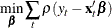where the loss functionMinimization of the loss functionis equivalent to the maximization of a likelihood function formed by combining independently distributed asymmetric Laplace densities (Yu and Moyeed, 2001).

The asymmetric Laplace distribution has the following probability density function (Yu and Zhang, 2005):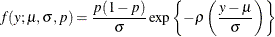Given the observations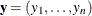, the posterior distribution of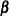,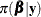is given by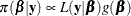where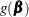is the prior distribution ofandis the likelihood function, written as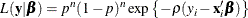There is no standard conjugate prior distribution for the parameters of the pth regression quantile, but you can use Markov chain Monte Carlo (MCMC) methods to approximate the posterior distributions of the unknown parameters. Yu and Moyeed (2001) prove that if you choose the prior ofto be improper uniform, then the resulting joint posterior distribution is proper.

## Fitting a Single Quantile Regression Function

This example from Yu and Moyeed (2001) explores the distribution of the serum concentration (grams per liter) of immunoglobulin-G (IgG) conditional on age in a sample of 298 children. A detailed discussion of the data appears in Isaacs et al. (1983) and Royston and Altman (1994). The following DATA step reads the data and creates the data set Igg:


data igg;
input IgG Age;
Age2=age*age;
datalines;
1.5         .5
2.7         .5
1.9         .5

... more lines ...

7.6   5.916667
3.1          6
6.8          6
;
run;


You can use the SGPLOT procedure as follows to generate a histogram of the response variable IgG for a visual inspection of its marginal distribution:


ods graphics on;
proc sgplot data=igg;
histogram igg / nbins=50;
run;


Figure 1 shows the distribution of IgG. The distribution is asymmetric and skewed to the right.

Figure 1: Distribution of IgGYou can also use PROC SGPLOT as follows to generate a scatterplot of IgG by Age:


proc sgplot data=igg;
scatter x=Age y=IgG;
run;


Figure 2 shows the relationship between IgG and Age. There is some evidence of an upward trend.

Figure 2: IgG by Age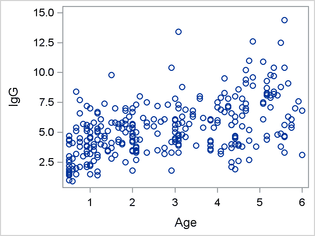Yu and Moyeed (2001) fit a quantile regression model of the formThe following SAS statements demonstrate how to use the MCMC procedure to fit this quantile regression model for a single quantile. Because PROC MCMC does not recognize the asymmetric Laplace distribution, you must write out the log-likelihood function and use the GENERAL() function.

The PROPCOV option in the PROC MCMC statement specifies that the conjugate-gradient method be used in constructing the initial covariance matrix for the Metropolis-Hastings algorithm. The NTU= option requests 1,000 tuning iterations. Experience shows that increasing the number of tuning iterations often results in improved mixing of the Markov chains. The MINTUNE= option requests a minimum of 10 proposal tuning loops. Increasing the minimum number of proposal tuning loops above the default number of 2 is sometimes needed in order to achieve convergence of the Markov chain. The NMC= option requests 30,000 MCMC iterations, excluding the burn-in iterations.

The BEGINCNST and ENDCNST statements define a statement block within which PROC MCMC processes the programming statements only during the setup stage of the simulation. You can use the BEGINCNST and ENDCNST statement block to define the constant, the quantile that is being fitted.

The PARMS statement lists the model parameters, b0, b1, and b2, and specifies that their initial values be 0.

The PRIOR statement specifies that the parameters b0, b1, and b2 have independent, improper uniform prior distributions.

The next three statements define the log-likelihood of the quantile regression model. The first and second statements are for convenience in writing the third statement. The first statement defines Mu, the location parameter of the asymmetric Laplace distribution. The second statement defines the random variable u, which is equal to the response variable IgG minus the location parameter Mu. The third statement defines the log-likelihood function in terms of the quantileand the random variable u.

The MODEL statement specifies that the response variable IgG have an asymmetric Laplace distribution, as defined by the log-likelihood function LL.


proc mcmc data=igg
seed=5263
propcov=congra
ntu=1000
mintune=10
nmc=30000;
begincnst;
p=0.95;
endcnst;
parms (b0-b2) 0;
prior b: ~ general(0);
mu= b0 + b1*age + b2*age2;
u = igg - mu;
ll = log(p)+log(1-p) - 0.5*(abs(u)+(2*p-1)*u);
model igg ~ general(ll);
run;


Figure 3 shows the trace plots, autocorrelation plots, and kernel density estimates of the parameters b0, b1, and b2, respectively. All three trace plots indicate convergence of the Markov chain. The autocorrelation plots show evidence of autocorrelation in the posterior samples, which often indicates slow mixing. The kernel density estimates are unimodal and relatively smooth.

Figure 3: PROC MCMC Diagnostic Plots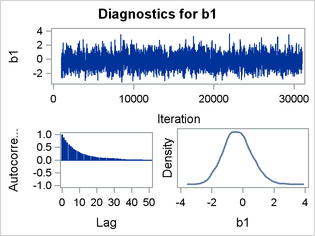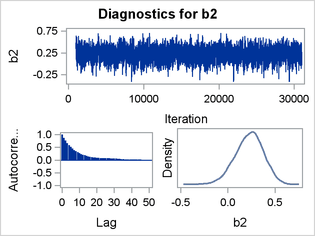Output 1 shows that the Monte Carlo standard errors (MCSE) of each parameter are small relative to the posterior standard deviations (SD). A small MCSE/SD ratio indicates that the Markov chain has stabilized and the mean estimates do not vary much over time.

Output 1: Monte Carlo Standard Errors

The MCMC Procedure

Monte Carlo Standard Errors
Parameter MCSE Standard
Deviation
MCSE/SD
b0 0.0307 1.1056 0.0277
b1 0.0245 0.9086 0.0269
b2 0.00350 0.1488 0.0235

Output 2 shows the Effective Sample Sizes table. The autocorrelation times for the three parameters range from 16.59 to 23.08, and the efficiency rates are low. These results account for the relatively small effective sample sizes, given a nominal sample size of 30,000.

Output 2: Effective Sample Size

Effective Sample Sizes
Parameter ESS Autocorrelation
Time
Efficiency
b0 1299.3 23.0889 0.0433
b1 1380.4 21.7326 0.0460
b2 1808.2 16.5906 0.0603

Output 3 and Output 4 show the posterior summaries of the estimated parameters b0, b1, and b2. The sample mean of the output chain for the intercept, b0, is 7.17. The 95% credible interval is strictly positive, indicating with high probability that the intercept is positive. The sample mean for b1, the coefficient of the variable Age, is –0.33. The 95% credible interval contains 0, indicating that the direction of the effect of Age on the 0.95 quantile is ambiguous. The sample mean for b2, the coefficient of Age2, is 0.23. The 95% credible interval also contains 0, indicating ambiguity about the direction of the effect of Age2 on the 0.95 quantile.

Output 3: Posterior Summaries

The MCMC Procedure

Posterior Summaries
Parameter N Mean Standard
Deviation
Percentiles
25% 50% 75%
b0 30000 7.1693 1.1056 6.4442 7.1752 7.9161
b1 30000 -0.3342 0.9086 -0.9559 -0.3713 0.2496
b2 30000 0.2263 0.1488 0.1291 0.2339 0.3292

Output 4: Posterior Intervals

Posterior Intervals
Parameter Alpha Equal-Tail Interval HPD Interval
b0 0.050 4.9733 9.3065 4.9718 9.2998
b1 0.050 -2.0134 1.5389 -2.1007 1.4119
b2 0.050 -0.0814 0.4997 -0.0614 0.5144

## Fitting a Quantile Process

In many quantile regression problems, it is useful to examine the quantile process, or how the estimated regression parameter for each covariate changes asvaries over the interval. This example, which is a variation on an example from Yu and Moyeed (2001), explores the stack loss data from Brownlee (1965). The data are from the operation of a plant where ammonia is oxidized to nitric acid; the oxidation is measured on 21 consecutive days. The response variable, StackLoss, is the percentage of ammonia lost (times 10), and there are three explanatory variables: AirFlow, which measures the air flow to the plant; WaterTemp, which measures the cooling water inlet temperature; and AcidConc, which measures the acid concentration. The following DATA step reads the data and creates a SAS data set:


data stackloss;
input stackloss airflow watertemp acidconc;
datalines;
42       80         27         89
37       80         27         88
37       75         25         90
28       62         24         87
18       62         22         87
18       62         23         87
19       62         24         93
20       62         24         93
15       58         23         87
14       58         18         80
14       58         18         89
13       58         17         88
11       58         18         82
12       58         19         93
8       50         18         89
7       50         18         86
8       50         19         72
8       50         19         79
9       50         20         80
15       56         20         82
15       70         20         91
;
run;


Figure 4 shows the distribution of StackLoss and scatter plots of StackLoss by AirFlow, StackLoss by WaterTemp, and StackLoss by AcidConc. The distribution of StackLoss is bimodal and right-skewed, and it has a noticeable gap between the values of 20 and 28. These characteristics suggest the possibility that the response variable has a mixture distribution.

The relationship between StackLoss and AirFlow appears to be linear. The relationship between StackLoss and WaterTemp is approximately linear, but there is a suggestion of nonlinearity. The relationship between StackLoss and AcidConc might best be described as heteroscedastic. Again, there is a suggestion that the response variable has a mixture distribution because of the apparent clustering of StackLoss as AcidConc increases.

Figure 4: Visual Inspection of the Data

 Distribution of Stack Loss Stack Loss by Air Flow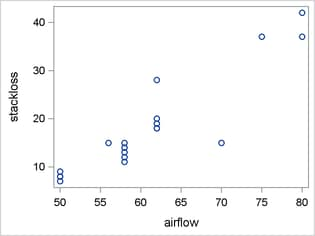Stack Loss by Water Temperature Stackloss by Acid Concentration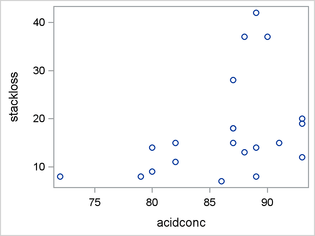Yu and Moyeed (2001) fit the following quantile regression model to these data: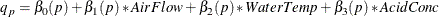The following statements show an efficient way to use the MCMC procedure to request the estimated quantile processes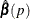for the quantile regression parameters. Suppose you span the range ofin increments of 0.05. That is, you want to estimate thequantiles; there are 19 quantiles in all. First, you create a new data set that has 19 copies of your original data set. The new data set must be indexed and sorted by. Second, you use the MCMC procedure’s BY-group processing capabilities to estimate the model parameters for each of the 19 quantiles. The ODS OUTPUT statement saves the Posterior Summaries and Posterior Intervals tables as SAS data sets. These data sets are used later to produce plots of the quantile processes for each parameter. The DATA= option of the PROC MCMC statement specifies the newly created input data set. The BEGINCNST and ENDCNST statement block, where you previously specified a single value for, is replaced with a BY statement. < /p>


data by_stackloss;
set stackloss;
do p = .05 to .95 by .05;
output;
end;
run;


proc sort data=by_stackloss;
by p;
run;


ods output postsummaries=by_ps postintervals=by_pi;
proc mcmc data=by_stackloss
seed=73625
propcov=congra
ntu=1000
nmc=30000
mintune=17;
by p;
parms (b0-b3) 0;
prior b: ~ general(0);
mu= b0 + b1*airflow + b2*watertemp + b3*acidconc;
u = stackloss - mu;
ll = log(p)+log(1-p) - 0.5*(abs(u)+(2*p-1)*u);
model stackloss ~ general(ll);
run;


The contents of the Posterior Summaries and Posterior Intervals tables for the whole quantile process are saved in the ODS OUTPUT data sets By_ps and By_pi, respectively. The following statements merge these two data sets, sort the merged data set, and then use the SGPLOT and PRINT procedures to produce quantile process plots and tables for each model parameter:


data process;
merge by_ps by_pi;
run;


proc sort data=process out=process;
by parameter p;
run;


proc sgplot data=process(where=(parameter="b0"));
title "Estimated Parameter by Quantile";
title2 "With 95% HPD Interval";
series x=p y=mean / markers legendlabel="Intercept (b0)";
band x=p lower=hpdlower upper=hpdupper /
transparency=.5 legendlabel="HPD Interval";
yaxis label="Intercept (b0)";
xaxis label="Quantile";
refline 0 / axis=y;
run;


proc print data=process(where=(parameter="b0")) noobs;
title "Estimated Parameter b0 by Quantile";
title2 "with 95% HPD Interval";
var p mean hpdlower hpdupper;
run;


Figure 5 and Output 5 show that the sample means for the intercept, b0, are negative throughout the process. They are increasing up to the 0.15 quantile and are then relatively constant up to the 0.60 quantile. The means then begin a steady decline until the 0.85 quantile, where they begin increasing. The 95% HPD intervals are negative throughout most the process; the exception is the 0.95 quantile, whose 95% HPD interval contains 0.

Figure 5: Quantile Process Plot for b0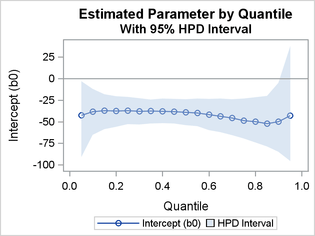Output 5: Quantile Process Table for b0

 Estimated Parameter b0 by Quantile with 95% HPD Interval

p Mean HPDLower HPDUpper
0.05 -42.3985 -90.8604 -3.2819
0.10 -38.1142 -65.1973 -11.9736
0.15 -36.9064 -58.7638 -18.1234
0.20 -37.2556 -55.8252 -20.4854
0.25 -37.2191 -52.7650 -21.1718
0.30 -37.9856 -53.1388 -22.6125
0.35 -37.2883 -52.2435 -24.4803
0.40 -37.6403 -51.7583 -23.0280
0.45 -38.0287 -52.0388 -23.5456
0.50 -38.8226 -54.0858 -24.0051
0.55 -39.9354 -55.3115 -23.0169
0.60 -41.6264 -59.8976 -23.6168
0.65 -43.4826 -62.0332 -23.2145
0.70 -45.5962 -65.6766 -23.9189
0.75 -48.4560 -69.5842 -23.1632
0.80 -49.8484 -73.9430 -21.7130
0.85 -51.8986 -78.5607 -20.1638
0.90 -49.9473 -84.8666 -4.8727
0.95 -42.9003 -95.6122 37.8042

Figure 6 and Output 6 show that the sample means for the parameter b1, the coefficient for AirFlow, are positive throughout the process. The means increase for most of the process, peak at the 0.65 quantile, decrease monotonically until the 0.90 quantile, and then increase slightly. The 95% HPD intervals are all strictly positive except for the 0.05 quantile’s interval, which contains 0.

Figure 6: Quantile Process Plot for b1Output 6: Quantile Process Table for b1

 Estimated Parameter b1 by Quantile with 95% HPD Interval
p Mean HPDLower HPDUpper
0.05 0.3885 -0.1160 0.8970
0.10 0.4493 0.1007 0.8213
0.15 0.5060 0.1859 0.8358
0.20 0.5871 0.2660 0.8972
0.25 0.6534 0.3645 0.9323
0.30 0.7105 0.4329 0.9787
0.35 0.7515 0.4798 0.9767
0.40 0.7873 0.5527 1.0260
0.45 0.8133 0.5935 1.0535
0.50 0.8315 0.5984 1.0586
0.55 0.8575 0.6314 1.0952
0.60 0.8752 0.6505 1.1113
0.65 0.8847 0.6481 1.1320
0.70 0.8800 0.6196 1.1402
0.75 0.8570 0.5780 1.1169
0.80 0.8391 0.5218 1.1347
0.85 0.7879 0.4486 1.1065
0.90 0.7624 0.3821 1.1625
0.95 0.7639 0.1712 1.4504

Figure 7 and Output 7 show that the sample means for the parameter b2, the coefficient for WaterTemp, are positive throughout the process. The means decrease monotonically up to the 0.50 quantile and then increase monotonically, forming a quadratic shape. The 95% HPD intervals are all strictly positive.

Figure 7: Quantile Process Plot for b2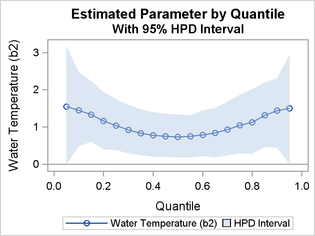Output 7: Quantile Process Table for b2
 Estimated Parameter b2 by Quantile with 95% HPD Interval

p Mean HPDLower HPDUpper
0.05 1.5434 -0.0237 3.1776
0.10 1.4464 0.4691 2.4739
0.15 1.3340 0.5991 2.2340
0.20 1.1584 0.3870 1.9407
0.25 1.0332 0.3694 1.7456
0.30 0.9197 0.2825 1.5866
0.35 0.8305 0.2426 1.4704
0.40 0.7735 0.1924 1.3922
0.45 0.7526 0.1880 1.3552
0.50 0.7331 0.1789 1.3296
0.55 0.7457 0.1715 1.3120
0.60 0.7871 0.2148 1.4364
0.65 0.8367 0.1736 1.5076
0.70 0.9299 0.2258 1.6847
0.75 1.0437 0.2869 1.8634
0.80 1.1216 0.2783 2.0078
0.85 1.3144 0.4507 2.1898
0.90 1.4403 0.4344 2.3049
0.95 1.4978 0.000710 2.9429

Figure 8 and Output 8 show that the sample means for the parameter b3, the coefficient for AcidConc, are relatively constant and close to, but systematically below, 0. The 95% HPD intervals contain 0 throughout the process.

Figure 8: Quantile Process Plot for b3Output 8: Quantile Process Table for b3
 Estimated Parameter b3 by Quantile with 95% HPD Interval

p Mean HPDLower HPDUpper
0.05 -0.0273 -0.6761 0.5518
0.10 -0.0742 -0.5038 0.3504
0.15 -0.0888 -0.4208 0.2613
0.20 -0.0902 -0.3912 0.1849
0.25 -0.0995 -0.3469 0.1370
0.30 -0.0975 -0.3252 0.1201
0.35 -0.1079 -0.3162 0.0850
0.40 -0.1109 -0.3057 0.0971
0.45 -0.1153 -0.3107 0.0875
0.50 -0.1101 -0.3099 0.0961
0.55 -0.1134 -0.3317 0.0889
0.60 -0.1106 -0.3472 0.1124
0.65 -0.1026 -0.3502 0.1337
0.70 -0.0917 -0.3749 0.1417
0.75 -0.0655 -0.3670 0.2100
0.80 -0.0501 -0.3994 0.2571
0.85 -0.0292 -0.4046 0.3260
0.90 -0.0539 -0.6201 0.4144
0.95 -0.1274 -0.9977 0.6559

## References

• Brownlee, K. A. (1965), Statistical Theory and Methodology in Science and Engineering, New York: John Wiley & Sons.

• Isaacs, D., Altman, D. G., Tidmarsh, C. E., Valman, H. B., and Webster, A. D. B. (1983), “Serum Immunoglobulin Concentrations in Preschool Children Measured by Laser Nephelometry: Reference Ranges for IgG, IgA, IgM,” Journal of Clinical Pathology, 36, 1193–1196.

• Royston, P. and Altman, D. G. (1994), “Regression Using Fractional Polynomials of Continuous Covariates: Parsimonious Parametric Modelling (with Discussion),” Applied Statistics, 43, 429–467.

• Yu, K. and Moyeed, R. A. (2001), “Bayesian Quantile Regression,” Statistics and Probability Letters, 54, 437–447.

• Yu, K. and Zhang, J. (2005), “A Three-Parameter Asymmetric Laplace Distribution and Its Extension,” Communications in Statistics—Theory and Methods, 34, 1867–1879.